# RD Sharma Solutions Class 9 Chapter 15 Area of Parallelograms and Triangles

Read RD Sharma Solutions Class 9 Chapter 15 Area of Parallelograms and Triangles below, students should study RD Sharma class 9 Mathematics available on Studiestoday.com with solved questions and answers. These chapter wise answers for class 9 Mathematics have been prepared by teacher of Grade 9. These RD Sharma class 9 Solutions have been designed as per the latest NCERT syllabus for class 9 and if practiced thoroughly can help you to score good marks in standard 9 Mathematics class tests and examinations

Exercise 15.1

Question 1: Which of the following figures lie on the same base and between the same parallel. In such a case, write the common base and two parallels: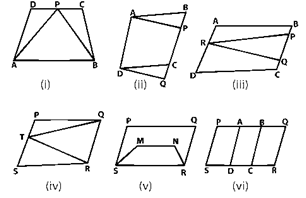Solution:
(i) ∆APB and trapezium ABCD are on the same base AB and between the same parallels AB and DC.
Here, Common base is AB and Parallel lines are AB and DC.

(ii) Parallelograms ABCD and APQD are on the common base AD and between the same parallels AD and BQ. Here, Common base is AD and Parallel lines are AD and BQ.

(iii) The base of parallelogram ABCD and ΔPQR is different but lies between the same parallels AD and BC.

(iv) ΔQRT and parallelogram PQRS are lies between same parallels QR and PS and on the same base QR.
Here, Common base is QR and Parallel lines are QR and PS.

(v) The base of parallelograms PQRS and trapezium SMNR are on common base SR, but not on same parallels.

(vi) PQRS, AQRD, BCQR parallelograms are between the same parallels.

Exercise 15.2

Question 1: If figure, ABCD is a parallelogram, AE ⊥ DC and CF ⊥ AD. If AB = 16 cm, AE = 8 cm and CF = 10 cm, find AD.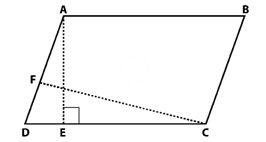Solution:
In parallelogram ABCD, AB = 16 cm, AE = 8 cm and CF = 10 cm
AE ⊥ DC
Area of parallelogram = Base × height
Area of parallelogram = Base × Altitude
Here DC is base and AE is the height.
Area of parallelogram ABCD = DC × AE
Area of parallelogram ABCD = 16 × 18
Area of parallelogram ABCD = 128cm
Here AD is base and CF is the height.
Area of parallelogram ABCD = AD x CF
Hence, the measure of AD is 12.8 cm

Question 2: In Q.No. 1, if AD = 6 cm, CF = 10 cm and AE = 8 cm, find AB.
Solution:
AE ⊥ DC
AB = DC
Area of parallelogram = Base × height
Area of a parallelogram ABCD = AD × CF
Area of a parallelogram ABCD = 6 × 10
Area of a parallelogram ABCD = 60

Area of a parallelogram ABCD = DC × AE
60 = CD × 8
60/8 = CD
CD = 7.5
AB = DC = 7.5 cm (opposite sides of a parallelogram are equal)

Question 3: Let ABCD be a parallelogram of area 124 cm2. If E and F are the mid-points of sides AB and CD respectively, then find the area of parallelogram AEFD.
Solution:
It is given that, ABCD be a parallelogram.
Area of parallelogram = 124 cm2
Area of parallelogram EBCF = FC x AP
Area of parallelogram AFED = DF x AP
Area of parallelogram AEFD = Area of parallelogram EBCF = 1/2 (Area of parallelogram ABCD)
Area of parallelogram AEFD = 124/2
Area of parallelogram AEFD = 62cm2
Hence, area of parallelogram AEFD is 62 cm2.

Question 4: If ABCD is a parallelogram, then prove that ar(ΔABD) = ar(Δ BCD) = ar(Δ ABC) = ar(Δ ACD) = 1/2 ar(||gm ABCD)
Solution:
AB is common base of parallelogram ABCD.
Construct diagonal AC and BD.
Let AC is the diagonal, then,
Area (ΔABC) = Area (ΔACD) = 1/2 (Area of llgm ABCD)__________(1)
Let BD be another diagonal
Area (ΔABD) = Area (ΔBCD) = 1/2 (Area of llgm ABCD) __________(2)
From equation (1) and (2) we get,
Area (ΔABC) = Area (ΔACD) = Area (ΔABD) = Area (ΔBCD) = 1/2 (Area of llgm ABCD)Hence Proved

Exercise 15.3
Question 1: In figure, compute the area of quadrilateral ABCD.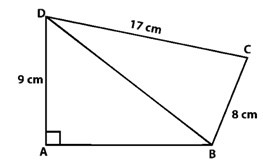Solution:
It is given that, ABCD is a quadrilateral
Here,
DC = 17 cm
BC = 8 cm
ΔBCD is a right triangle,
CD2 =  BD2 + BC2 (By Pythagorean Theorem)
172 =  BD2 + 82
289 =  BD2 + 64
BD2 = 289 – 64
BD2 = 225
BD = √225
BD = 15
ΔABD is a right triangle,
BD2= AB2 + AD2 (By Pythagorean Theorem)
152 = AB2 + 92
225 = AB2 + 92
AB= 225−81
AB2 = 144
AB = 12
Area of ΔABD = 1/2 (12 × 9) cm2
Area of ΔABD = 54 cm2
Area of ΔBCD = 1/2 (8 × 17) cm
Area of ΔBCD = 68 cm2
Area of quadrilateral ABCD = Area of ΔABD + Area of ΔBCD
Area of quadrilateral ABCD = 54 cm2 + 68 cm2
Area of quadrilateral ABCD = 112 cm2
Hence, the area of quadrilateral ABCD is 112 cm2.

Question 2: In figure, PQRS is a square and T and U are, respectively, the mid-points of PS and QR . Find the area of ΔOTS if PQ = 8 cm.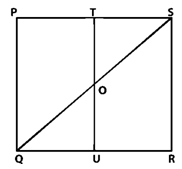Solution:
It is given that, T and U are mid points of PS and QR respectively.
TU||PQ
TO||PQ
In ΔPQS,
T is the mid-point of PS and TO||PQ
TO = 1/2 PQ (by mid-point theorem)
TO = 1/2 (8) (Given PQ is 8 cm)
TO = 4 cm
TS = 1/2 PS
TS = 1/2 (8) (Given PS is 8 cm)
TS = 4 cm
PQ = PS, As PQRS is a square
Area of ΔOTS = 1/2 (TO × TS)
Area of ΔOTS = 1/2(4 × 4) cm2
Area of ΔOTS = 8cm2
Hence, the area of ΔOTS is 8 cm2.

Question 3: Compute the area of trapezium PQRS in figure.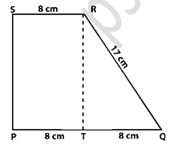Solution:
Area of trapezium PQRS = Area of rectangle PSRT + Area of ΔQRT
Area of trapezium PQRS = (PT × RT) + 1/2 (QT × RT)
Area of trapezium PQRS = (8 × RT) + 1/2 (8 × RT)
Area of trapezium PQRS = 8RT + 8RT/2
Area of trapezium PQRS = (16RT+8RT)/2
Area of trapezium PQRS = 24RT/2
Area of trapezium PQRS = 12RT
ΔQRT is a right triangle,
OR2 = QT2 + RT2 (Using Pythagorean Theorem)
RT2 = QR2 − QT2
RT2 = 172 − 82
RT2  = 225
RT = 15
Area of trapezium = 12 × 15 cm
Area of trapezium = 180 cm2
Hence, the area of trapezium is 180 cm2.

Question 4: In figure, ∠AOB = 90o, AC = BC, OA = 12 cm and OC = 6.5 cm. Find the area of ΔAOB.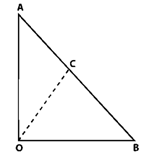Solution:
It is given that, AOB is a triangle.
∠AOB = 90°
AC = BC, OA = 12cm
OC = 6.5cm
As we know, the midpoint of the hypotenuse of a right triangle is equidistant from the vertices.
CB = CA = OC = 6.5 cm
AB = 2 CB
AB = 2 x 6.5cm
AB = 13cm
ΔOAB is a right triangle:
AB2 = OB2 + OA2 (Using Pythagorean Theorem)
132 = OB2 + 122
OB2 = 169 – 144
OB2 = 25
OB = 5cm
Area of ΔAOB = 1/2 (Base × height) cm
Area of ΔAOB = 1/2 (12 x 5) cm2
Area of ΔAOB = 30cm2
Hence, the area of ΔAOB is 30cm2.

Question 5: In figure, ABCD is a trapezium in which AB = 7 cm, AD = BC = 5 cm, DC = x cm, and distance between AB and DC is 4 cm. Find the value of x and area of trapezium ABCD.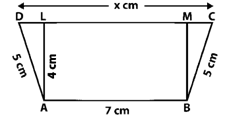Solution:
It is given that, ABCD is a trapezium
AB = 7 cm
AD = BC = 5 cm
DC = x cm
Distance between AB and DC = 4 cm
Consider AL and BM are perpendiculars on DC, then
AL = BM = 4 cm and LM = 7 cm.
ΔBMC is a right triangle,
BC2 = BM2 + MC2 (Pythagorean Theorem)
52 = 42 + MC2
25 = 16 + MC2
MC2 = 25 – 16
MC= 9
MC = 3
AD2 = AL2 + DL2 (Pythagorean Theorem)
52 = 42 + DL2
25 = 16 + DL2
DL2 = 25 – 16 = 9
DL = 3
Thus,
x = DC = DL + LM + MC
x = 3 + 7 + 3 = 13
x = 13 cm
Area of trapezium ABCD = 1/2 (AB + CD) AL
Area of trapezium ABCD = 1/2(7+13)×4
Area of trapezium ABCD = 40cm
Hence, the area of trapezium ABCD is 40 cm2.

Question 6: In figure, OCDE is a rectangle inscribed in a quadrant of a circle of radius 10 cm. If OE = 2√5 cm, find the area of the rectangle.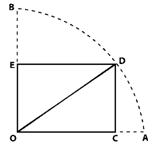Solution:
It is given that,
Radius = OD = 10 cm and OE = 2√5 cm
In right ΔDEO,
By Pythagoras theorem
OD2 = OE2 + DE2
(10)2 = (2√5  )2 + DE2
100 – 20 = DE2
DE = 4√5
Area of rectangle = Length x Breadth
Area of rectangle OCDE = OE x DE
Area of rectangle OCDE = 2√5 x 4√5
Area of rectangle OCDE = 40
Hence, the area of rectangle is 40 cm2.

Question 7: In figure, ABCD is a trapezium in which AB || DC. Prove that ar(ΔAOD) = ar(ΔBOC)Solution:
It is given that, ABCD is a trapezium in which AB || DC.
We can observe that ΔADC and ΔBDC are sharing common base DC and between same parallels AB and DC.
ΔADC is the combination of triangles, ΔAOD and ΔDOC.
ΔBDC is the combination of ΔBOC and ΔDOC.
From the equation (1)
ar(ΔAOD) + ar(ΔDOC) = ar(ΔBOC) + ar(ΔDOC)
ar(ΔAOD) = ar(ΔBOC)
Hence Proved

Question 8: In figure, ABCD, ABFE and CDEF are parallelograms. Prove that ar(ΔADE) = ar(ΔBCF).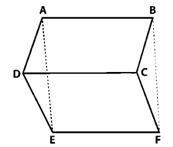Solution:
It is given that, ABCD, CDEF and ABFE are parallelograms.
DE = CF
AE = BF
DE = CF (Proved above)
AE = BF (Proved above)
ΔADE ≅ ΔBCF (By SSS criterion of congruence)
Hence Proved

Question 9: Diagonals AC and BD of a quadrilateral ABCD intersect each other at P. Show that: ar(ΔAPB) × ar(ΔCPD) = ar(ΔAPD) × ar(ΔBPC).
Solution:
It is given that, diagonals AC and BD of a quadrilateral ABCD intersect each other at P.
BQ and DR are two perpendiculars on AC.
ar(ΔAPB) × ar(ΔCDP) = ar(ΔAPD) × ar(ΔBPC)
(1/2×AP×BQ) × (1/2×PC×DR) = ar(ΔAPD) × ar(ΔBPC)
(1/2×PC×BQ) × (1/2×AP×DR) = ar(ΔAPD) × ar(ΔBPC)
ar(ΔAPD) x ar(ΔBPC) = ar(ΔAPD) × ar(ΔBPC)
L.H.S. = R.H.S.
Hence proved

Question 10: In figure, ABC and ABD are two triangles on the base AB. If line segment CD is bisected by AB at O, show that ar(ΔABC) = ar(ΔABD).Solution:
Draw two perpendiculars CP and DQ on AB.
ar(ΔABC) = 1/2 × AB × CP__________(1)
ar(ΔABD) = 1/2×AB×DQ __________ (2)
In triangles, ΔCPO and ΔDQO
∠CPO = ∠DQO (each 90°)
CO = OD (Given)
∠COP = ∠DOQ (Vertically opposite angles)
ΔCP0 ≅ ΔDQO (By AAS condition)
CP = DQ _________(3) (CPCT)
From equations (1), (2) and (3)
ar(ΔABC) = ar(ΔABD)
Hence proved

Question 11:  If P is any point in the interior of a parallelogram ABCD, then prove that area of the triangle APB is less than half the area of parallelogram.
Solution:
Construct DN ⊥ AB and PM ⊥ AB
ar(∥gm ABCD) = AB × DN,
ar(ΔAPB) = 1/2(AB × PM)
PM < DN
AB × PM < AB × DN
1/2 (AB × PM) < 1/2 (AB × DN)
ar(ΔAPB) < 1/2 ar (∥gm ABCD)
Hence Proved

Question 12:  If AD is a median of a triangle ABC, then prove that triangles ADB and ADC are equal in area. If G is the mid-point of the median AD, prove that ar(ΔBGC) = 2ar(ΔAGC).
Solution:
It is given that, AD is the median of ΔABC
Construct AM ⊥ BC
BD = DC__________(1)
Multiply AM on both sides of equation (1)
BD × AM = DC × AM
1/2 (BD × AM) = 1/2 (DC × AM)
ar(ΔABD) = ar(ΔACD) _________(2)

In ΔBGC, GD is the median
ar(ΔBGD) = ar(ΔCGD) _________ (3)

In ΔACD, CG is the median
ar(ΔAGC) = ar(ΔCGD) _________ (4)

From (3) and (4) we get,
ar(ΔBGD) = ar(ΔAGC)
ar(ΔBGC) = 2ar(ΔBGD)
ar(ΔBGC) = 2ar(ΔAGC)
Hence Proved

Question 13:  A point D is taken on the side BC of a ΔABC, such that BD = 2DC. Prove that ar(ΔABD) = 2ar(ΔADC).
Solution:
It is given that, In ΔABC, BD = 2DC
Take a point E on BD such that BE = ED
BE = ED = DC
We know that median of triangle divides it into two equal triangles.
In ΔABD,
AE is the median.
ar(ΔABD) = 2ar(ΔAED)_________(1)
In ΔAEC,
From equation (1) and (2) we get,
Hence proved

Question 14: ABCD is a parallelogram whose diagonal intersects at O .If P is any point on BO, prove that:
(ii) ar(ΔABP) = 2ar(ΔCBP).
Solution:
It is given that, ABCD is the parallelogram.
We know that diagonals of parallelogram bisect each other
AO = OC and BO = OD
(i) In ΔDAC,
DO is a median.

(ii) In ΔBAC,
BO is a median.
ar(ΔBAO) = ar(ΔBCO) ________(1)
In ΔPAC,
PO is a median.
ar(ΔPAO) = ar(ΔPCO) ________ (2)
Subtract equation 2 from 1.
ar(ΔBAO) − ar(ΔPAO) = ar(ΔBCO) − ar(ΔPCO)
ar(ΔABP) = 2ar(ΔCBP).
Hence proved

Question 15:  ABCD is a parallelogram in which BC is produced to E such that CE = BC. AE intersects CD at F.
(i) Prove that ar(ΔADF) = ar(ΔECF).
(ii) If the area of ΔDFB = 3 cm2, find the area of ∥gm ABCD.
Solution:
∠ADF = ∠ECF (Alternate interior angles)
∠DFA = ∠CFA  (Vertically opposite angles)
ΔADF ≅ ΔECF (by AAS congruence criterion)
DF = CF (CPCT)
DF = CF
BF is a median in Δ BCD
ar(ΔBCD) = 2ar(ΔBDF)
ar(ΔBCD) = 2 × 3 cm
ar(ΔBCD) = 6 cm2
Area of a parallelogram = 2 × ar(ΔBCD)
Area of a parallelogram = 2 × 6cm
Area of a parallelogram = 12cm2
Hence, the area of parallelogram of is 12cm2.

Question 16: ABCD is a parallelogram whose diagonals AC and BD intersect at O. A line through O intersects AB at P and DC at Q. Prove that ar(ΔPOA) = ar(ΔQOC).
Solution: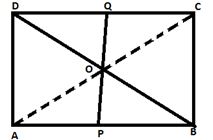In ∆OAP and ∆OQC
∠AOP = ∠QOC (Vertically opposite angle)
AO = OC (opposite sides of a ||gm)
∠PAC = ∠QCA (Alternate interior angle)
ΔOAP ≅ ΔOQC (by ASA congruence criterion)
ar(ΔPOA) = ar(ΔQOC)

Question 17:  ABCD is a parallelogram. E is a point on BA such that BE = 2EA and F is point on DC such that DF = 2FC. Prove that AECF is a parallelogram whose area is one third of the area of parallelogram ABCD.
Solution: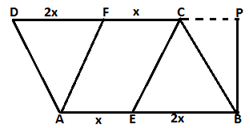AB = DC = 3x
AB = DC = 3AE
Now,
AE = FC = 1/3 of AB
AE || FC as they lies between AB and DC.
AECF is a parallelogram.
ar||gm AECF = AE × BP
ar||gm ABCD = AB × BP
Put the value of AB.
ar||gm ABCD = 3AE × BP
we know that AE × BP is ar||gm AECF.
ar||gm ABCD = 3 ar||gm AECF

Question 18:  In a triangle ABC, P and Q are respectively the mid points of AB and BC and R is the midpoint of AP. Prove that:
(i) ar(ΔPBQ) = ar(ΔARC).
(ii) ar(ΔPRQ) = 1/2ar(ΔARC).
(iii) ar(ΔRQC) = 3/8ar(ΔABC).
Solution:
(i) In ΔCAP
CR is the median
ar(ΔCRA) = 1/2ar(ΔCAP) ________(1)
In Δ CAB
CP is the median
ar(ΔCAP) = ar(ΔCPB) ________ (2)
From equation (1) and (2)
ar(ΔARC) = 1/2ar(ΔCPB) ________ (3)
In ΔPBC
PQ is the median
ar(ΔCPB) = 2ar(ΔPBQ)________(4)
From equation (3) and (4)
ar(ΔARC) = ar(ΔPBQ)_________(5)

(ii) In ∆QAB QP is the median and in ∆QAP QR is the median
ar(ΔQAP) = ar(ΔQBP)________(6)
ar(ΔQAP) = 2ar(ΔQRP) ________ (7)
From equation (6) and (7)
ar(ΔPRQ) = 1/2ar(ΔPBQ) ________ (8)
From equation (5) and (8)
ar(ΔPRQ) = 1/2ar(ΔARC)

(iii) In ΔCAP
LR is the median
ar(ΔARC) = 1/2 × 1/2 ar(ΔABC)
ar(ΔARC) = 1/4 ar(ΔABC)
In ΔRBC
RQ is the median
ar(ΔRQC) = 1/2 ar(ΔRBC)
ar(ΔRQC) = 1/2 {ar(ΔABC) − ar(ΔARC)}
ar(ΔRQC) = 1/2 {ar(ΔABC) – 1/4 ar(ΔABC)
ar(ΔRQC) = 3/8 ar(ΔABC)

Question 19:  ABCD is a parallelogram. G is a point on AB such that AG = 2GB and E is point on DC such that CE = 2DE and F is the point of BC such that BF = 2FC. Prove that:
(ii) ar(ΔEGB) = 1/6 ar(ABCD)
(iii) ar(ΔEFC) = 1/2 ar(ΔEBF)
(iv) ar(ΔEGB) = 3/2 × ar(ΔEFC)
(v) Find what portion of the area of parallelogram is the area of ΔEFG.
Solution:
It is given that ABCD is a parallelogram
AG = 2 GB, CE = 2 DE and BF = 2 FC
Construct a parallel line to AB through point F and a perpendicular line to AB from C(i) ABCD is a parallelogram
So, AB = CD and AD = BC
From two trapeziums ADEG and GBCE, we get
AB = DC, EC = 2DE, AG = 2GB
ED = 1/3 CD = 1/3 AB
EC = 2/3 CD = 2/3 AB
AG = 2/3 AB
BG = 1/3 AB
DE + AG = 1/3 AB + 2/3 AB
DE + AG = (1AB+2AB)/3
DE + AG = 3AB/3
DE + AG = AB
EC + BG = 2/3 AB + 1/3 AB
EC + BG = (2AB+1AB)/3
EC + BG = 3AB/3
EC + BG = AB
Trapezium ADEG and GBCE have same height and sum of two parallel sides are equal
Area of trapezium = (Sum of Parallel sides)/2×height

(ii) From above we know
BG = 1/2AB
ar(ΔEGB) = 1/2 × GB × Height
ar(ΔEGB) = 1/2 × 1/3 × AB × Height
ar(ΔEGB) = 1/6 × AB × Height
ar(ΔEGB) = 1/6ar(ABCD)

(iii) Since height if triangle EFC and EBF are equal. So
ar(ΔEFC) = 1/2 × FC × Height
ar(ΔEFC) = 1/2 × 1/2 × FB × Height
ar(ΔEFC) = 1/2 ar(∆EBF)
Hence, the ar(ΔEFC) is 1/2ar(ΔEBF)

(iv) ar(EGBC) = ar(ΔEGB) + ar(ΔEBF) + ar(ΔEFC)
1/2 ar(ABCD) = 1/6 ar(ABCD) + 2ar(ΔEFC) + ar(ΔEFC)
1/3 ar(ABCD) = 3 ar(ΔEFC)
ar(ΔEFC) = 1/9 ar(ABCD)
From equation (2) we get,
ar(ΔEGB) = 1/6 ar(ΔEFC)
ar(ΔEGB) = 3/2 × 1/9 ar(ABCD)
ar(ΔEGB) = 3/2 ar(ΔEFC)
ar(ΔEGB) = 3/2 ar(ΔEFC)

(v) It is given that FB = 2CF
Let CF = x
FB = 2x.
Now consider the two triangles CFI and CBH which are similar triangle.
So by the property of similar triangle CI = k and IH = 2k
Now consider the triangle EGF in which
ar(ΔEFG) = ar(ΔESF) + ar(ΔSGF)
ar(ΔEFG) = 1/2 SF × k + 1/2 SF × 2k
ar(ΔEFG) = 3/2 SF × k_________(i)
ar(ΔEGBC) = ar(SGBF) + ar(ESFC)
ar(ΔEGBC) = 1/2 (SF + GB) × 2k + 1/2 (SF + EC) × k
ar(ΔEGBC) = 3/2k × SF + (GB + (1/2)EC) × k
ar(ΔEGBC) = 3/2k × SF + (1/3 AB + 1/2 × 2/3 AB) × k
1/2 ar(ΔABCD) = 3/2 k × SF + 2/3 AB × k
ar(ΔABCD) = 3k × SF + (4/3) AB × k (Multiply both sides by 2)
ar(ΔABCD) = 3k × SF + 4/9 ar(ABCD)
k × SF = 5/27ar(ABCD) ⋅⋅⋅⋅  (2)
From equation (1) and (2) we get,
ar(ΔEFG) = 3/2 × 5/27 ar(ABCD)
ar(ΔEFG) = 5/18 ar(ABCD)
Hence, the area of ∆EFG is 5/18 ar(ABCD)

Question 20:  In figure, CD ∥ AE and CY ∥ BA.
(i) Name a triangle equal in area of ΔCBX
(ii) Prove that ar(ΔZDE) = ar(ΔCZA)
(iii) Prove that ar(BCZY) = ar(ΔEDZ)Solution:
(i) CY is common base
ar∆CAY = ar∆CYB
∆XYC + ∆XYA = ∆XYC + ∆BXC
ar∆CBX = ar∆XYA
Hence, the triangle equal in area of ΔCBX is ∆XYA.

(ii) CD is common base
ar∆CDA = ar∆CDE
∆CDZ + ∆CZA = ∆CDZ + ∆DZE
ar∆CZA = ar∆ZDE
Hence Prove

(iii) CY is Common base
ar∆CAY + ∆CYZ = ar∆CYB + ∆CYZ
ar∆CZA = ar∆BCZY
ar∆ZDE = ar∆BCZY
Hence Prove

Question 21:  In figure, PSDA is a parallelogram in which PQ = QR = RS and AP ∥ BQ ∥ CR. Prove that ar (ΔPQE) = ar(ΔCFD).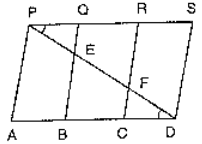Solution:
It is given that, PSDA is a parallelogram
AP ∥ BQ ∥ CR ∥ DS and AD ∥ PS
PQ = CD_______(1)
In ∆BED
C is the midpoint of BD
CF ∥ BE
F is the midpoint of ED
EF = PE
In ∆PQE and ∆CFD, we have
PE = FD (proved above)
∠ EPQ = ∠FDC (Alternate angles)
EF = PE
ΔPQE ≅ ΔDCF (by SAS criterion)
ar(ΔPQE) = ar(ΔDCF)
Hence Proved

Question 22:  In figure, ABCD is a trapezium in which AB || DC and DC = 40 cm and AB = 60 cm .If X and Y are, respectively, the mid points of AD and BC, prove that:
(i) XY = 50 cm
(ii) DCYX is a trapezium
(iii) ar(trap. DCYX) = 9/11 ar(XYBA).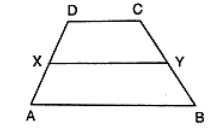Solution:
(i) DY and produce it to meet AB produced at P.
In ∆BYP and ∆CYD we have,
∠BYP = ∠ CYD (Vertically opposite angles)
∠DCY = ∠ PBY (DC ∥ AP)
BY = CY (Y is the mid-point)
(ΔBYP) ≅ (ΔCYD) (by ASA congruence criterion)
DY = YP (CPCT)
DC = BP (CPCT)
Y is the midpoint of DP
x is the midpoint of AD
XY ∥ AP and XY ∥ 1/2 AP
XY = 1/2 (AB + BP)
XY = 1/2 (AB + DC)
XY = 1/2 (60 + 40)
XY = 50 cm

(ii) We have, XY ∥ AP
XY ∥ AB and AB ∥ DC
XY ∥ DC
DCYX is a trapezium

(iii) x and y are the mid points of AD and BC.
Trapezium DCYX and ABYX are of the same height
ar(trap. DCXY) = 1/2(DC + XY) × h
ar(trap. DCXY) = 1/2 (50 + 40) × h cm
ar(trap. DCXY) = 45 h cm2

ar(trap. ABYX) = 1/2 (AB + XY) × h
ar(trap. ABYX) = 1/2 (60 + 50) × h cm
ar(trap. ABYX) = 55h cm2
(ar(trap.DCYX))/(ar(trap.ABYX))=45h/55h
(ar(trap.DCYX))/(ar(trap.ABYX))=9/11
ar(trap. DCYX) = 9/11 ar(trap. ABYX)
Hence, the ar(trap. DCYX) is 9/11 ar(trap. ABYX)

Question 23:  In figure ABC and BDE are two equilateral triangles such that D is the midpoint of BC. AE intersects BC in F. Prove that: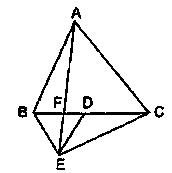(i) ar(ΔBDE) = 1/4 ar(ΔABC)
(ii) ar(ΔBDE) = 1/2ar(ΔBAE)
(iii) ar(ΔBFE) = ar(ΔAFD)
(iv) ar(ΔABC) = 2 ar(ΔBEC)
(v) ar(ΔFED) = 1/8 ar(ΔAFC)
(vi) ar(ΔBFE) = 2 ar(ΔEFD)
Solution:
It is given that ∆ABC and ∆BDE are two equilateral triangles.
Let AB = BC = CA = x. Then, BD = x/2 = DE = BE
(i) ar(∆ABC) = √3/4 x2
ar(∆BDE) = √3/4 (x/2)2
ar(∆BDE) = √3/4× (x2/4)
Hence, ar(ΔBDE) = 1/4 ar(ΔABC)

(ii) It is given that triangles, ABC and BED are equilateral triangles
∠ ACB = ∠DBE (each 60°)
BE ∥ AC (Alternative angles are equal)
∆BAF and ∆BEC are on the same base BE and between same parallels BF and AC.
ar(ΔBAE) = ar(ΔBEC)
ar(ΔBAE) = 2ar(ΔBDE) (ED is a median of triangle ∆EBC)
ar(ΔBDE) = 1/2ar(ΔBAE)

(iii) ∆ABC and ∆BDE are equilateral triangles
∠ABC = 60°
∠BDE = 60°
∠ABC = ∠BDE
AB ∥ DE (alternate angles are equal)
∆BED and ∆AED are on the same base ED and between same parallels AB and DE.
ar(ΔBED) = ar(ΔAED)
ar(ΔBED) − ar(ΔEFD) = ar(ΔAED) − ar(ΔEFD)
ar(ΔBEF) = ar(ΔAFD)

(iv) ED is the median of triangle BEC
ar(ΔBEC) = 2ar(ΔBDE)
ar(ΔBEC) = 2 × 1/2ar(ΔABC) (ar(ΔBDE) = 1/2 ar(ΔABC)]
ar(ΔBEC) = 1/2ar(ΔABC)
ar(ΔABC) = 2ar(ΔBEC)

(v) ar(ΔAFC) = ar(ΔAFD) + ar(ΔADC)
ar(ΔBFE) + 1/2ar(ΔABC)
We know that
ar(ΔBFE) + 1/2 × 4ar(ΔBDE)
ar(ΔBFE) = 2ar(ΔFED) _______(3)
ar(ΔBDE) = ar(ΔBFE) + ar(ΔFED)
2ar(ΔFED) + ar(ΔFED)
3ar(ΔFED)________(4)
From equation (2), (3) and (4) we get,
ar(ΔAFC) = 2ar(ΔFED) + 2 × 3 ar(ΔFED)
ar(ΔAFC) = 8 ar(ΔFED)
ar(ΔFED) = 1/8ar(ΔAFC)

(vi) Let us assumed that ‘H’ be the height of vertex A and ‘h’ be the height of vertex E
From part (i) we get,
ar(ΔBDE) = 1/4 ar(ΔABC)
1/2 × BD × h = 1/4 (1/2 × BC × h)
BD × h = 1/4 (2BD × H)
h = 1/2H _______(1)
From part (iii), we have
ar(ΔBFE) = ar(ΔAFD)
ar(ΔBFE) = 1/2 × FD × H
ar(ΔBFE) = 1/2 × FD × 2h
ar(ΔBFE) = 2((1/2) × FD × h)
ar(ΔBFE) = 2ar(ΔEFD)

Question 24:  D is the midpoint of side BC of ΔABC and E is the midpoint of BD. If O is the midpoint of AE, Prove that ar(ΔBOE) = 1/8 ar(ΔABC).
Solution: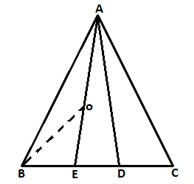It is given that, D is the midpoint of sides BC of ∆ABC
In ∆ABC
ar∆ABC = 1/2ar∆ABC
E is the mid-point
ar∆ABE = ar∆AED
ar∆ABE = 1/2×1/2ar∆ABC
ar∆ABE = 1/4ar∆ABC
In ∆ABE
O is the mid-point
ar∆ABO = ar∆OBE
ar∆ABO = 1/2 × 1/4ar∆ABC
ar∆ABO = 1/8ar∆ABC
Hence Proved

Question 25:  In figure, X and Y are the mid points of AC and AB respectively, QP ∥ BC and CYQ and BXP are straight lines. Prove that ar(ΔABP) = ar(ΔACQ).Solution:
X is the mid-point of AC and Y is AB
XY ∥ BC
∆BYC and ∆BXC are on the same base BC and between the same parallels XY and BC
ar(ΔBYC) = ar(ΔBXC)
ar(ΔBYC) − ar(ΔBOC) = ar(ΔBXC) − ar(ΔBOC)
ar(ΔBOY) = ar(ΔCOX)
ar(ΔBOY) + ar(ΔXOY) = ar(ΔCOX) + ar(ΔXOY)
ar(ΔBXY) = ar(ΔCXY)_________(1)
ar(quad. XYAP) = ar(quad. XYQA) (Quadrilaterals XYAP and XYAQ are on the same base XY and between same parallels XY and PQ)
By adding (1) and (2), we get
ar(ΔABP) = ar(ΔACQ)
Hence Proved

Question 26:  In figure, ABCD and AEFD are two parallelograms. Prove that
(i) PE = FQ
(ii) ar(ΔAPE) : ar(ΔPFA) = ar(ΔQFD):  ar(ΔPFD)
(iii) ar(ΔPEA) = ar(ΔQFD)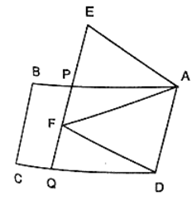Solution:
It is given that, ABCD and AEFD are two parallelograms
(i) In ∆EPA and ∆FQD
∠PEA = ∠QFD (corresponding angles)
∠EPA = ∠FQD (corresponding angles)
PA = QD (opposite sides of parallelogram)
ΔEPA ≅ ΔFQD (By AAS condition)
EP = FQ (C.P.C.T)

(ii) ∆PEA and ∆QFD stand on equal bases PE and FQ lies between the same parallels EQ and AD
ar(ΔPEA) = ar(ΔQFD)_______(1)
∆PEA and ∆PFD stand on the same base PF and between same parallels PF and AD
ar(ΔPFA) = ar(ΔPFD) _______ (2)
Divide the equation 1 by equation 2
(ar(ΔPEA))/(ar(ΔPFA)) = (ar(ΔQFD))/(ar(ΔPFD))
ar(ΔAPE) : ar(ΔPFA) = ar(ΔQFD):  ar(ΔPFD)
Hence Proved

(iii) We know that from part (i),
ΔEPA ≅ ΔFQD
ar(ΔPEA) =  ar(ΔQFD)

Question 27:  In figure, ABCD is a parallelogram. O is any point on AC. PQ ∥  AB and LM ∥ AD. Prove that: ar(∥gm DLOP) = ar(∥gm BMOQ).Solution:
We know that, a diagonal of a parallelogram divides it into two triangles of equal area
ar(ΔAPO) + ar(∥gm DLOP) + ar(ΔOLC) = ar(ΔAOM) + ar(∥gm BMOQ) + ar(ΔOQC) __________(1)
AO and OC are diagonals of parallelograms AMOP and OQCL
ar(ΔAPO) = ar(ΔAMO) ______(2)
ar(ΔOLC) = ar(ΔOQC) _______(3)
By subtracting equation (2) and (3) from (1)
ar(∥gm DLOP) = ar(∥gm BMOQ).

Question 28:  In a triangle ABC, if L and M are points on AB and AC respectively such that LM ∥ BC. Prove that:
(i) ar(ΔLCM) = ar(ΔLBM)
(ii) ar(ΔLBC) = ar(ΔMBC)
(iii) ar(ΔABM) = ar(ΔACL)
(iv) ar(ΔLOB) = ar(ΔMOC)
Solution:(i) In ∆LMB and ∆LMC are on the same base LM and between the same parallels LM and BC.
ar(ΔLMB) = ar(ΔLMC)
Hence Proved

(ii) ∆LBC and ∆MBC are on the same base BC and between same parallels LM and BC.
ar(ΔLBC) = ar(ΔMBC)
Hence Proved

(iii) We know that, ar(ΔLMB) = ar(ΔLMC)
ar(ΔALM) + ar(ΔLMB) = ar(ΔALM) + ar(ΔLMC)
ar(ΔABM) = ar(ΔACL)
Hence Proved

(iv) We know that, ar(ΔLBC) = ar(ΔMBC)
ar(ΔLBC) − ar(ΔBOC) = ar(ΔMBC) − ar(ΔBOC)
ar(ΔLOB) = ar(ΔMOC)
Hence Proved

Question 29:  In figure, D and E are two points on BC such that BD = DE = EC. Show that ar(ΔABD) = ar(ΔADE) = ar(ΔAEC).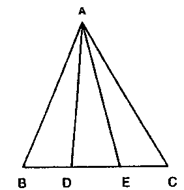Solution:
Draw a line l through A parallel to BC.
It is given that, BD = DE = EC
∆ABD and ∆AEC are on the equal bases and between the same parallels l and BC.
Hence Proved.

Question: 30  In figure, ABC is a right angled triangle at A, BCED, ACFG and ABMN are squares on the sides BC, CA and AB respectively. Line segment AX ⊥ DE meets BC at Y. Show that
(i) ΔMBC ≅ ΔABD
(ii) ar(BYXD) = 2ar(ΔMBC)
(iii) ar(BYXD) = ar(ABMN)
(iv) ΔFCB ≅ ΔACE
(v) ar(CYXE) = 2ar(ΔFCB)
(vi) ar(CYXE) = ar(ACFG)
(vii) ar(BCED) = ar(ABMN) + ar(ACFG)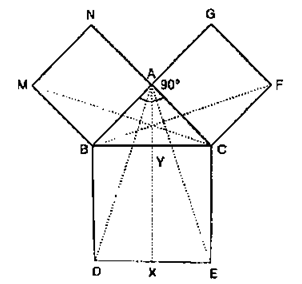Solution:
(i) In ΔMBC and ΔABD
MB = AB
BC = BD
∠MBC = ∠ABD (∠ABC to a right angle)
ΔMBC ≅ ΔABD (by SAS congruence criterion)
ar(ΔMBC) = ar(ΔABD)

(ii) ∆ABC and rectangle BYXD are on the same base BD and between the same parallels AX and BD.
ar(ΔABD) = 1/2 ar(rect BYXD)
ar(rect BYXD) = 2ar(ΔABD)
ar(rect BYXD) = 2ar(ΔMBC)

(iii) ∆MBC and square MBAN are on the same base MB and between the same parallels MB and NC.
2ar(ΔMBC) = ar(MBAN)
From equation (2) and (3)
ar(sq. MBAN) = ar(rect BYXD)

(iv) ∆FCB and ∆ACE
FC = AC
CB = CE
∠FCB = ∠ACE (∠ACB to a right angle)
ΔFCB ≅ ΔACE (by SAS congruence criterion)

(v) We know that,
ΔFCB ≅ ΔACE
ar(ΔFCB) = ar(ΔACE)
∆ACE and rectangle CYXE are on the same base CE and between same parallels CE and AX.
2ar(ΔACE) = ar(CYXE)
2ar(ΔFCB) = ar(ΔCYXE)

(vi) ∆FCB and rectangle FCAG are on the same base FC and between the same parallels FC and BG.
2ar(ΔFCB) = ar(FCAG)
ar(CYXE) = ar(ACFG)
Hence proved

(vii) BC2 = AB2 + AC2 (By Pythagoras theorem in triangle ACB)
BC × BD = AB × MB + AC × FC
ar(BCED) = ar(ABMN) + ar(ACFG)

Exercise VSAQs .......................

Question 1: If ABC and BDE are two equilateral triangles such that D is the mid-point of BC, then find ar(△ABC) : ar(△BDE).
Solution:
It is given ∆ABC and ∆BDE are two equilateral triangles.
Area of an equilateral triangle = √3/4 (side)2
Let us assumed that the side of a triangle is ‘a’.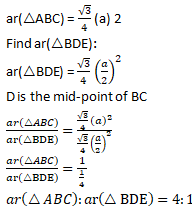Question 2: In figure, ABCD is a rectangle in which CD = 6 cm, AD = 8 cm. Find the area of parallelogram CDEF.Solution:
It is given that, ABCD is a rectangle
Where CD = 6 cm and AD = 8 cm
llgm CDEF and rectangle ABCD on the same base and between the same parallels, which means both have equal areas.
Area of parallelogram CDEF = Area of rectangle ABCD
Area of rectangle ABCD = CD x AD
Area of rectangle ABCD = 6 x 8 cm2
Area of rectangle ABCD = 48 cm2

Question 3: In figure, find the area of ΔGEF.Solution:
Parallelogram CDEF and rectangle ABCD on the same base and between the same parallels.
Area of CDEF = Area of ABCD = 8 x 6 cm2
Area of CDEF = Area of ABCD = 48 cm2
llgm CDEF and ∆EFG are on the same base and between the same parallels, then
Area of a triangle = 1/2 (Area of parallelogram)
Area of a triangle EFG = 1/2 (Area of parallelogram CDEF) = 1/2 (48 cm2
Area of a triangle EFG = 1/2 (Area of parallelogram CDEF) = 24 cm2.

Question 4: In figure, ABCD is a rectangle with sides AB = 10 cm and AD = 5 cm. Find the area of ΔEFG.Solution:
Area of ABEF = Area of ABCD
Area of ABEF = Area of ABCD = 10 x 5 cm2
Area of ABEF = Area of ABCD = 50 cm2
Parallelogram ABEF and triangle EFG are on the same base and between the same parallels, then
Area of a triangle = 1/2 (Area of parallelogram)
Area of a triangle EFG = 1/2 (Area of parallelogram ABEF)
Area of a triangle EFG = 1/2 (Area of parallelogram ABEF) = 1/2 (50 cm2
Area of a triangle EFG = 1/2 (Area of parallelogram ABEF) = 25 cm2.

Question 5: PQRS is a rectangle inscribed in a quadrant of a circle of radius 13 cm. A is any point on PQ. If PS = 5 cm, then find ar(△RAS).
Solution:
It is given that PQRS is a rectangle
PS = 5cm
PR = 13cm
In ∆PSR
SR2 = PR2 – PS2 (Using Pythagoras theorem)
SR2=(13)2 – (5)2
SR2  = 169 – 25
SR2  = 114
SR = 12
Area of △RAS = 1/2 x SR x PS
Area of △RAS = 1/2 x 12 x 5
Area of △RAS = 30
Thus, Area of △RAS is 30 cm2.